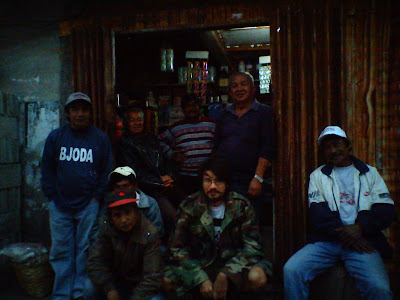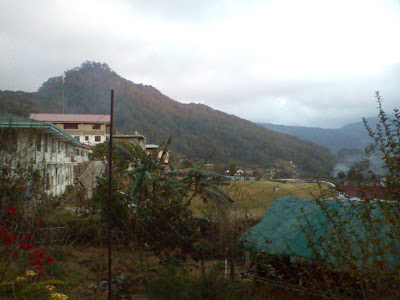## Wednesday, January 23, 2013

### Mountain Province

I have been in the Philippines for the past several years, most of that time has been in north western Mountain Province - homeland of the Kankanaey Igorots. Soon I will be traveling back to L.A. California.### Photo of Me, Salude Pekas with her grandchildren: Troy and Shaun.### Photo of Me with "Cadiogan's Regulars" in Besao### Photo of Muguy Mountain in Besao### "LaUnion Fish" - oil-stick and pastels on canvas board## Sunday, January 20, 2013

### import_blend.py

Jeroen Bakker has a excellent article about Blender's binary file format and its DNA structure here, he is also the author or Blender-Aid, that contains a script for loading and saving .blend files. Unfortunately the loading script is not ideal for general use: ie. it only supports some data structures, and is missing support for array data. It is also licensed using the GNU GPL, making it incompatible with closed source software.

Last week I started work on a new .blend importer that works with Python2 and Python3, this is licensed using the "New BSD" license so it can be used by anyone without "copyleft" restrictions. The new importer supports: basic arrays, multi-dimensional arrays, nested structures, pointers, and lazy unpacking of attributes.

### Parsing .blend

Blender saves by dumping the scene directly from memory to a .blend file; together with a minimal header and the "DNA" footer.

Parsing a .blend file has three initial steps: 1. reading the file header to get the pointer size and endianess, 2. reading all data blocks, 3. unpacking the "DNA1" block at the end. The special DNA1 block is a structure that contains a description of all C Struct types that Blender saves in a .blend file. Using this information you can unpack the all the other blocks into Python objects. Data blocks can vary in size depending on the host machine that saved the .blend file, this is because different CPU types and different Operating Systems can have different endianess, pointer and integer sizes.

### Using import_blend.py

Using import_blend you can directly access the raw DNA structures. If you familiar with Blender's Python API, it is similar, but not the same, for example when getting the name of an object it is prefixed by "OB" if its an Object or "CA" if its a Camera data object.
```import import_blend

blenderfile = import_blend.load( '/path/some.blend' )
ob = blenderfile.database['Object']
print(ob)
print('-'*80)
print('object name: ' + ob.id.name)
print('data name: ' + ob.data.id.name)
```

#### output from the above example

```≺Object:
id              (ID  100)             = ≺Object::ID at 147229548≻
sculpt          (*SculptSession  0)
type            (short  2)            = 11
partype         (short  2)            = 0
par1            (int  4)              = 0
par2            (int  4)              = 0
par3            (int  4)              = 0
parsubstr       (char  1) array:  = u''
parent          (*Object  1164)
track           (*Object  1164)
proxy           (*Object  1164)
proxy_group     (*Object  1164)
proxy_from      (*Object  1164)
ipo             (*Ipo  132)
bb              (*BoundBox  104)
action          (*bAction  148)
poselib         (*bAction  148)
pose            (*bPose  188)
data            (*void  0)                    = ≺Object::Camera at 146475340≻
gpd             (*bGPdata  120)
avs             (bAnimVizSettings  48)        = ≺Object::bAnimVizSettings at 3075066700≻
mpath           (*bMotionPath  20)
constraintChannels(ListBase  8)               = ≺Object::ListBase at 3075065804≻
effect          (ListBase  8)         = ≺Object::ListBase at 147229100≻
disp            (ListBase  8)         = ≺Object::ListBase at 147227308≻
defbase         (ListBase  8)         = ≺Object::ListBase at 147227116≻
modifiers       (ListBase  8)         = ≺Object::ListBase at 147243084≻
mode            (int  4)              = 0
restore_mode    (int  4)              = 0
mat             (*Material  768)
matbits         (*char  1)
totcol          (int  4)              = 0
actcol          (int  4)              = 0
loc             (float  4) array:          = [7.481131553649902, -6.5076398849487305, 5.34366512298584]
dloc            (float  4) array:          = [0.0, 0.0, 0.0]
orig            (float  4) array:          = [0.0, 0.0, 0.0]
size            (float  4) array:          = [1.0, 1.0, 1.0]
dsize           (float  4) array:          = [0.0, 0.0, 0.0]
dscale          (float  4) array:          = [1.0, 1.0, 1.0]
rot             (float  4) array:          = [1.1093189716339111, 0.010816991329193115, 0.8149281740188599]
drot            (float  4) array:          = [0.0, 0.0, 0.0]
quat            (float  4) array:          = [1.0, 0.0, 0.0, 0.0]
dquat           (float  4) array:          = [1.0, 0.0, 0.0, 0.0]
rotAxis         (float  4) array:          = [0.0, 1.0, 0.0]
drotAxis        (float  4) array:          = [0.0, 1.0, 0.0]
rotAngle        (float  4)            = 0.0
drotAngle       (float  4)            = 0.0
obmat           (float  4) array:[4, 4]               = [[0.6858805418014526, 0.6858805418014526, 0.6858805418014526, 0.6858805418014526], [0.6858805418014526, 0.7276337742805481, -0.010816780850291252, 0.0], [0.6858805418014526, -0.010816780850291252, -0.31737011671066284, 0.8953432440757751], [0.6858805418014526, 0.0, 0.8953432440757751, -0.6106656193733215]]
parentinv       (float  4) array:[4, 4]               = [[1.0, 1.0, 1.0, 1.0], [1.0, 0.0, 0.0, 0.0], [1.0, 0.0, 0.0, 0.0], [1.0, 0.0, 0.0, 0.0]]
constinv        (float  4) array:[4, 4]               = [[1.0000001192092896, 1.0000001192092896, 1.0000001192092896, 1.0000001192092896], [1.0000001192092896, 5.960465188081798e-08, -2.980232594040899e-08, 0.0], [1.0000001192092896, -2.980232594040899e-08, 2.9802322387695312e-08, 5.960465188081798e-08], [1.0000001192092896, 0.0, 5.960465188081798e-08, -1.776357262916724e-15]]
imat            (float  4) array:[4, 4]               = [[0.6858804821968079, 0.6858804821968079, 0.6858804821968079, 0.6858804821968079], [0.6858804821968079, -0.31737011671066284, 0.6548619270324707, 0.0], [0.6858804821968079, 0.6548619270324707, 0.7276336550712585, -0.6106656193733215], [0.6858804821968079, 0.0, -0.6106656193733215, 0.8953432440757751]]
imat_ren        (float  4) array:[4, 4]               = [[0.0, 0.0, 0.0, 0.0], [0.0, 0.0, 0.0, 0.0], [0.0, 0.0, 0.0, 0.0], [0.0, 0.0, 0.0, 0.0]]
lay             (int  4)              = 1
sf              (float  4)            = 0.0
flag            (short  2)            = 1024
colbits         (short  2)            = 0
transflag       (short  2)            = 0
protectflag     (short  2)            = 0
trackflag       (short  2)            = 5
upflag          (short  2)            = 1
nlaflag         (short  2)            = 0
ipoflag         (short  2)            = 0
scaflag         (short  2)            = 0
scavisflag      (char  1)             = u''
pad5            (char  1)             = u''
dupon           (int  4)              = 1
dupoff          (int  4)              = 0
dupsta          (int  4)              = 1
dupend          (int  4)              = 100
mass            (float  4)            = 1.0
damping         (float  4)            = 0.5659443736076355
inertia         (float  4)            = 1.0
formfactor      (float  4)            = 0.4000000059604645
rdamping        (float  4)            = 0.6258381009101868
sizefac         (float  4)            = 0.0
margin          (float  4)            = 0.05999999865889549
max_vel         (float  4)            = 0.0
min_vel         (float  4)            = 0.0
m_contactProcessingThreshold(float  4) = 0.0
obstacleRad     (float  4)            = 0.0
step_height     (float  4)            = 0.0
jump_speed      (float  4)            = 0.0
fall_speed      (float  4)            = 0.0
pad1            (char  1) array:   = u''
rotmode         (short  2)            = 1
boundtype       (char  1)             = u''
collision_boundtype(char  1)          = u''
restrictflag    (char  1)             = u''
dt              (char  1)             = u'\x02'
dtx             (char  1)             = u''
empty_drawtype  (char  1)             = u'\x01'
empty_drawsize  (float  4)            = 1.0
dupfacesca      (float  4)            = 1.0
prop            (ListBase  8)         = ≺Object::ListBase at 147245132≻
sensors         (ListBase  8)         = ≺Object::ListBase at 147246380≻
controllers     (ListBase  8)         = ≺Object::ListBase at 147226796≻
actuators       (ListBase  8)         = ≺Object::ListBase at 3075066828≻
bbsize          (float  4) array:  = [0.0, 0.0, 0.0]
index           (short  2)            = 0
actdef          (short  2)            = 0
col             (float  4) array:  = [0.0, 0.0, 0.0, 0.0]
gameflag        (int  4)              = 65536
gameflag2       (int  4)              = 0
bsoft           (*BulletSoftBody  120)
softflag        (short  2)            = 0
recalc          (short  2)            = 0
anisotropicFriction(float  4) array: = [1.0, 1.0, 1.0]
constraints     (ListBase  8)         = ≺Object::ListBase at 147218348≻
nlastrips       (ListBase  8)         = ≺Object::ListBase at 147243404≻
hooks           (ListBase  8)         = ≺Object::ListBase at 147229356≻
particlesystem  (ListBase  8)         = ≺Object::ListBase at 147244364≻
pd              (*PartDeflect  144)   = ≺Object::PartDeflect at 146476108≻
soft            (*SoftBody  440)
dup_group       (*Group  124)
body_type       (char  1)             = u''
shapeflag       (char  1)             = u''
shapenr         (short  2)            = 0
smoothresh      (float  4)            = 0.0
fluidsimSettings(*FluidsimSettings  1228)
derivedDeform   (*DerivedMesh  0)
derivedFinal    (*DerivedMesh  0)
lastDataMask    (uint64_t  8)         = 0
customdata_mask (uint64_t  8)         = 0
state           (int  4)              = 1
init_state      (int  4)              = 0
gpulamp         (ListBase  8)         = ≺Object::ListBase at 147229484≻
pc_ids          (ListBase  8)         = ≺Object::ListBase at 147244780≻
duplilist       (*ListBase  8)
ima_ofs         (float  4) array:  = [0.0, 0.0]
≻
--------------------------------------------------------------------------------
object name: OBCamera
data name: CACamera

```

## Sunday, January 13, 2013

### Automatic Object Orientation

C programs are often written in an "object oriented style" where functions take a pointer to a typedef'ed struct as the first argument. Using this huristic and modifying PyCParser's AST in place, we can reconstruct a Cython class that has proper methods. This provides an automated way to convert a C API into clean object oriented API.

### Example C API

```// basic typedef struct
typedef struct A {
int x;
float y;
} A;

void set_x( A *a, int x ) {
a->x = x;
}

int get_x( A *a ) {
return a->x;
}
```

### Automatic Object Orientation

```cdef class A:
cdef public int x
cdef public float y

def __init__(self, int x,float y):
self.x = x
self.y = y

cpdef void set_x(self, int x):
self.x = x

cpdef int get_x(self):
return self.x
```

source code

## Friday, January 11, 2013

### Automatic C to Cython Translator

Blender is too large to rewrite in Cython entirely by hand. I am now working on a c-to-cython translator using Eli Bendersky's PyCParser. Eli already has a working c-to-c translator that I am using as the starting point for c-to-cython.

### Fibonacci Series in C

```void main()
{
int n, first = 0, second = 1, next, c;
n = 1000;
printf("First %d terms of Fibonacci series are :-\n",n);
for ( c = 0 ; c < n ; c++ )
{
if ( c <= 1 )
next = c;
else
{
next = first + second;
first = second;
second = next;
}
printf("%d\n",next);
}
return 0;
}
```

### Fibonacci Series Automatically Translated to Cython

```cpdef void main():
cdef int n;
cdef int first = 0;
cdef int second = 1;
cdef int next;
cdef int c;
n = 1000;
print("First %d terms of Fibonacci series are :-\n", n);
for c from 0 <= c < n:
if (c <= 1):
next = c;
else:
next = first + second;
first = second;
second = next;
print("%d\n", next);
return 0;
```

### Typedef Structures - C

```// basic typedef struct
typedef struct A {
int x;
float y;
} A;

// struct with different typedef name
typedef struct Foo {
int z;
} Bar;

// basic struct
struct B {
int w;
};
```

### Typedef Structures - Automatically Translated to Cython

```ctypedef struct A:
int x
float y

cdef struct Foo:
int z
ctypedef Foo Bar

cdef struct B:
int w
```
c-to-cython source code (work in progress)

### Intro to BioBlender by Ashley Lee

Blender is being used for scientific visualization, one of the first projects was BioBlender. With BioBlender scientists can load protein databank files and render animations for visualization. See Intuitive representation of surface properties of biomolecules using BioBlender by Andrei et al. BMC Bioinformatics 2012 here.

### Zoppè M. et al. Vedere l'invisibile.

Blender is ideal for data visualization because of its powerful rendering features like: volumetric materials, point clouds, smoke and fluid dynamics, and fast GPU rendering. Reza Fatahi on the BF-Python mailing list is interested in using Blender to automate rendering of confocal microscopy files. Blender can also import data from Paraview.